# plot3

3-D point or line plot

•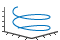## Syntax

``plot3(X,Y,Z)``
``plot3(X,Y,Z,LineSpec)``
``plot3(X1,Y1,Z1,...,Xn,Yn,Zn)``
``plot3(X1,Y1,Z1,LineSpec1,...,Xn,Yn,Zn,LineSpecn)``
``plot3(tbl,xvar,yvar,zvar)``
``plot3(ax,___)``
``plot3(___,Name,Value)``
``p = plot3(___)``

## Description

### Vector and Matrix Data

example

````plot3(X,Y,Z)` plots coordinates in 3-D space. To plot a set of coordinates connected by line segments, specify `X`, `Y`, and `Z` as vectors of the same length.To plot multiple sets of coordinates on the same set of axes, specify at least one of `X`, `Y`, or `Z` as a matrix and the others as vectors. ```

example

````plot3(X,Y,Z,LineSpec)` creates the plot using the specified line style, marker, and color.```

example

````plot3(X1,Y1,Z1,...,Xn,Yn,Zn)` plots multiple sets of coordinates on the same set of axes. Use this syntax as an alternative to specifying multiple sets as matrices.```

example

````plot3(X1,Y1,Z1,LineSpec1,...,Xn,Yn,Zn,LineSpecn)` assigns specific line styles, markers, and colors to each `XYZ` triplet. You can specify `LineSpec` for some triplets and omit it for others. For example, `plot3(X1,Y1,Z1,'o',X2,Y2,Z2)` specifies markers for the first triplet but not for the second triplet.```

### Table Data

example

````plot3(tbl,xvar,yvar,zvar)` plots the variables `xvar`, `yvar`, and `zvar` from the table `tbl`. To plot one data set, specify one variable each for `xvar`, `yvar`, and `zvar`. To plot multiple data sets, specify multiple variables for at least one of those arguments. The arguments that specify multiple variables must specify the same number of variables. (since R2022a)```

example

````plot3(ax,___)` displays the plot in the target axes. Specify the axes as the first argument in any of the previous syntaxes.```

example

````plot3(___,Name,Value)` specifies `Line` properties using one or more name-value pair arguments. Specify the properties after all other input arguments. For a list of properties, see Line Properties.```

example

````p = plot3(___)` returns a `Line` object or an array of `Line` objects. Use `p` to modify properties of the plot after creating it. For a list of properties, see Line Properties.```

## Examples

collapse all

Define `t` as a vector of values between 0 and 10$\pi$. Define `st` and `ct` as vectors of sine and cosine values. Then plot `st`, `ct`, and `t`.

```t = 0:pi/50:10*pi; st = sin(t); ct = cos(t); plot3(st,ct,t)```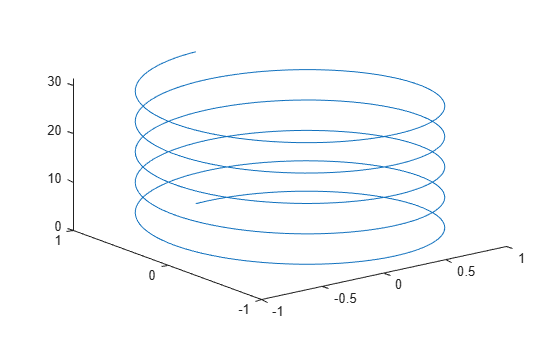Create two sets of x-, y-, and z-coordinates.

```t = 0:pi/500:pi; xt1 = sin(t).*cos(10*t); yt1 = sin(t).*sin(10*t); zt1 = cos(t); xt2 = sin(t).*cos(12*t); yt2 = sin(t).*sin(12*t); zt2 = cos(t);```

Call the `plot3` function, and specify consecutive `XYZ` triplets.

`plot3(xt1,yt1,zt1,xt2,yt2,zt2)`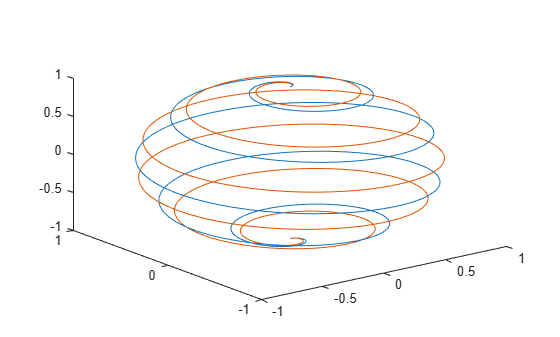Create matrix `X` containing three rows of x-coordinates. Create matrix `Y` containing three rows of y-coordinates.

```t = 0:pi/500:pi; X(1,:) = sin(t).*cos(10*t); X(2,:) = sin(t).*cos(12*t); X(3,:) = sin(t).*cos(20*t); Y(1,:) = sin(t).*sin(10*t); Y(2,:) = sin(t).*sin(12*t); Y(3,:) = sin(t).*sin(20*t);```

Create matrix `Z` containing the z-coordinates for all three sets.

`Z = cos(t);`

Plot all three sets of coordinates on the same set of axes.

`plot3(X,Y,Z)`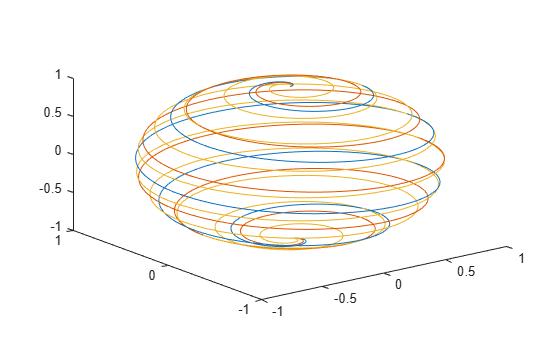Create vectors `xt`, `yt`, and `zt`.

```t = 0:pi/500:40*pi; xt = (3 + cos(sqrt(32)*t)).*cos(t); yt = sin(sqrt(32) * t); zt = (3 + cos(sqrt(32)*t)).*sin(t);```

Plot the data, and use the `axis equal` command to space the tick units equally along each axis. Then specify the labels for each axis.

```plot3(xt,yt,zt) axis equal xlabel('x(t)') ylabel('y(t)') zlabel('z(t)')```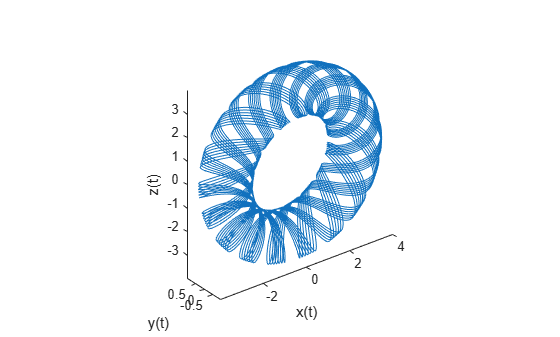Create vectors `t`, `xt`, and `yt`, and plot the points in those vectors using circular markers.

```t = 0:pi/20:10*pi; xt = sin(t); yt = cos(t); plot3(xt,yt,t,'o')```Create vectors `t`, `xt`, and `yt`, and plot the points in those vectors as a blue line with 10-point circular markers. Use a hexadecimal color code to specify a light blue fill color for the markers.

```t = 0:pi/20:10*pi; xt = sin(t); yt = cos(t); plot3(xt,yt,t,'-o','Color','b','MarkerSize',10,... 'MarkerFaceColor','#D9FFFF')```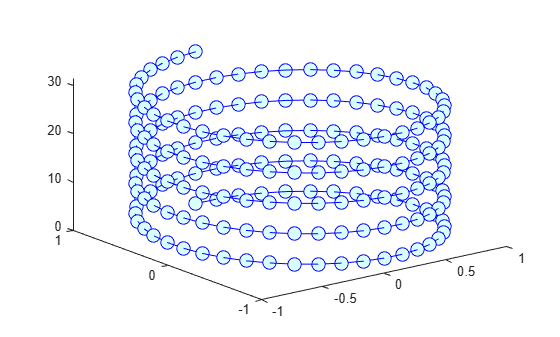Create vector `t`. Then use `t` to calculate two sets of x and y values.

```t = 0:pi/20:10*pi; xt1 = sin(t); yt1 = cos(t); xt2 = sin(2*t); yt2 = cos(2*t);```

Plot the two sets of values. Use the default line for the first set, and specify a dashed line for the second set.

`plot3(xt1,yt1,t,xt2,yt2,t,'--')`Create vectors `t`, `xt`, and `yt`, and plot the data in those vectors. Return the chart line in the output variable `p`.

```t = linspace(-10,10,1000); xt = exp(-t./10).*sin(5*t); yt = exp(-t./10).*cos(5*t); p = plot3(xt,yt,t);```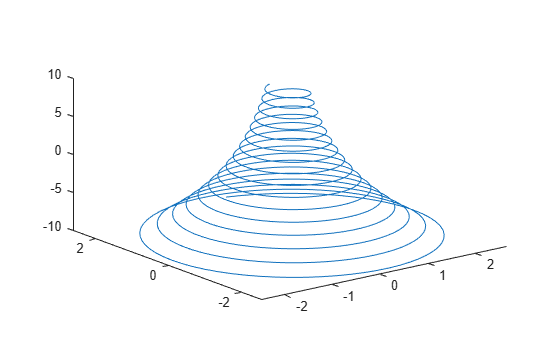Change the line width to `3`.

`p.LineWidth = 3;`Since R2022a

A convenient way to plot data from a table is to pass the table to the `plot3` function and specify the variables to plot.

Create vectors `x`, `y`, and `t`, and put the vectors in a table. Then display the first three rows of the table.

```t = (0:pi/20:10*pi)'; x = sin(t); y = cos(t); tbl = table(x,y,t); head(tbl,3)```
``` x y t _______ _______ _______ 0 1 0 0.15643 0.98769 0.15708 0.30902 0.95106 0.31416 ```

Plot the `x`, `y`, and `t` table variables. Return the `Line` object as `p`. Notice that the axis labels match the variable names.

`p = plot3(tbl,"x","y","t");`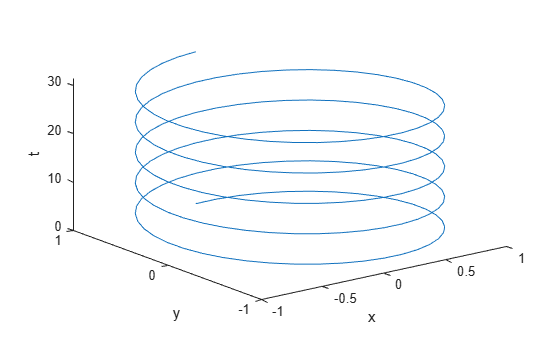To modify aspects of the line, set the `LineStyle`, `Color`, and `Marker` properties on the `Line` object. For example, change the line to a red dotted line with circular markers.

```p.LineStyle = ":"; p.Color = "red"; p.Marker = "o";```Since R2022a

Create a table containing five variables. Then display the first three rows of the table.

```t = (0:pi/500:pi)'; x1 = sin(t).*cos(10*t); x2 = sin(t).*cos(12*t); y1 = sin(t).*sin(10*t); y2 = sin(t).*sin(12*t); z = cos(t); tbl = table(x1,x2,y1,y2,z); head(tbl,3)```
``` x1 x2 y1 y2 z _________ _________ __________ __________ _______ 0 0 0 0 1 0.0062707 0.0062653 0.00039452 0.00047329 0.99998 0.012467 0.012423 0.0015749 0.0018877 0.99992 ```

Plot the `x1` and `x2` variables on the x-axis, the `y1` and `y2` variables on the y-axis, and the `z` variable on the z-axis. Then add a legend. Notice that the legend entries match the variable names.

```plot3(tbl,["x1","x2"],["y1","y2"],"z") legend```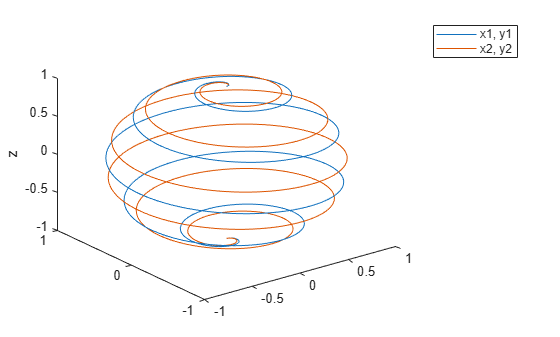Starting in R2019b, you can display a tiling of plots using the `tiledlayout` and `nexttile` functions. Call the `tiledlayout` function to create a 1-by-2 tiled chart layout. Call the `nexttile` function to create the axes objects `ax1` and `ax2`. Create separate line plots in the axes by specifying the axes object as the first argument to `plot`3.

```tiledlayout(1,2) % Left plot ax1 = nexttile; t = 0:pi/20:10*pi; xt1 = sin(t); yt1 = cos(t); plot3(ax1,xt1,yt1,t) title(ax1,'Helix With 5 Turns') % Right plot ax2 = nexttile; t = 0:pi/20:10*pi; xt2 = sin(2*t); yt2 = cos(2*t); plot3(ax2,xt2,yt2,t) title(ax2,'Helix With 10 Turns')```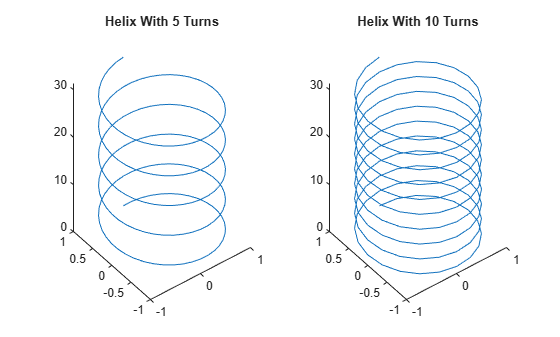Create `x` and `y` as vectors of random values between `0` and `1`. Create `z` as a vector of random duration values.

```x = rand(1,10); y = rand(1,10); z = duration(rand(10,1),randi(60,10,1),randi(60,10,1));```

Plot `x`, `y`, and `z`, and specify the format for the z-axis as minutes and seconds. Then add axis labels, and turn on the grid to make it easier to visualize the points within the plot box.

```plot3(x,y,z,'o','DurationTickFormat','mm:ss') xlabel('X') ylabel('Y') zlabel('Duration') grid on```Create vectors `xt`, `yt`, and `zt`. Plot the values, specifying a solid line with circular markers using the `LineSpec` argument. Specify the `MarkerIndices` property to place one marker at the 200th data point.

```t = 0:pi/500:pi; xt(1,:) = sin(t).*cos(10*t); yt(1,:) = sin(t).*sin(10*t); zt = cos(t); plot3(xt,yt,zt,'-o','MarkerIndices',200)```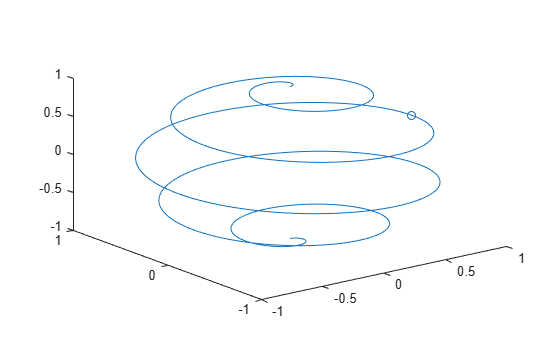## Input Arguments

collapse all

x-coordinates, specified as a scalar, vector, or matrix. The size and shape of `X` depends on the shape of your data and the type of plot you want to create. This table describes the most common situations.

Type of PlotHow to Specify Coordinates
Single point

Specify `X`, `Y`, and `Z` as scalars and include a marker. For example:

`plot3(1,2,3,'o')`

One set of points

Specify `X`, `Y`, and `Z` as any combination of row or column vectors of the same length. For example:

`plot3([1 2 3],[4; 5; 6],[7 8 9])`

Multiple sets of points
(using vectors)

Specify consecutive sets of `X`, `Y`, and `Z` vectors. For example:

`plot3([1 2 3],[4 5 6],[7 8 9],[1 2 3],[4 5 6],[10 11 12])`

Multiple sets of points
(using matrices)

Specify at least one of `X`, `Y`, or `Z` as a matrix, and the others as vectors. Each of `X`, `Y`, and `Z` must have at least one dimension that is same size. For best results, specify all vectors of the same shape and all matrices of the same shape. For example:

`plot3([1 2 3],[4 5 6],[7 8 9; 10 11 12])`

Data Types: `single` | `double` | `int8` | `int16` | `int32` | `int64` | `uint8` | `uint16` | `uint32` | `uint64` | `categorical` | `datetime` | `duration`

y-coordinates, specified as a scalar, vector, or matrix. The size and shape of `Y` depends on the shape of your data and the type of plot you want to create. This table describes the most common situations.

Type of PlotHow to Specify Coordinates
Single point

Specify `X`, `Y`, and `Z` as scalars and include a marker. For example:

`plot3(1,2,3,'o')`

One set of points

Specify `X`, `Y`, and `Z` as any combination of row or column vectors of the same length. For example:

`plot3([1 2 3],[4; 5; 6],[7 8 9])`

Multiple sets of points
(using vectors)

Specify consecutive sets of `X`, `Y`, and `Z` vectors. For example:

`plot3([1 2 3],[4 5 6],[7 8 9],[1 2 3],[4 5 6],[10 11 12])`

Multiple sets of points
(using matrices)

Specify at least one of `X`, `Y`, or `Z` as a matrix, and the others as vectors. Each of `X`, `Y`, and `Z` must have at least one dimension that is same size. For best results, specify all vectors of the same shape and all matrices of the same shape. For example:

`plot3([1 2 3],[4 5 6],[7 8 9; 10 11 12])`

Data Types: `single` | `double` | `int8` | `int16` | `int32` | `int64` | `uint8` | `uint16` | `uint32` | `uint64` | `categorical` | `datetime` | `duration`

z-coordinates, specified as a scalar, vector, or matrix. The size and shape of `Z` depends on the shape of your data and the type of plot you want to create. This table describes the most common situations.

Type of PlotHow to Specify Coordinates
Single point

Specify `X`, `Y`, and `Z` as scalars and include a marker. For example:

`plot3(1,2,3,'o')`

One set of points

Specify `X`, `Y`, and `Z` as any combination of row or column vectors of the same length. For example:

`plot3([1 2 3],[4; 5; 6],[7 8 9])`

Multiple sets of points
(using vectors)

Specify consecutive sets of `X`, `Y`, and `Z` vectors. For example:

`plot3([1 2 3],[4 5 6],[7 8 9],[1 2 3],[4 5 6],[10 11 12])`

Multiple sets of points
(using matrices)

Specify at least one of `X`, `Y`, or `Z` as a matrix, and the others as vectors. Each of `X`, `Y`, and `Z` must have at least one dimension that is same size. For best results, specify all vectors of the same shape and all matrices of the same shape. For example:

`plot3([1 2 3],[4 5 6],[7 8 9; 10 11 12])`

Data Types: `single` | `double` | `int8` | `int16` | `int32` | `int64` | `uint8` | `uint16` | `uint32` | `uint64` | `categorical` | `datetime` | `duration`

Line style, marker, and color, specified as a string scalar or character vector containing symbols. The symbols can appear in any order. You do not need to specify all three characteristics (line style, marker, and color). For example, if you omit the line style and specify the marker, then the plot shows only the marker and no line.

Example: `"--or"` is a red dashed line with circle markers.

Line StyleDescriptionResulting Line
`"-"`Solid line`"--"`Dashed line`":"`Dotted line`"-."`Dash-dotted lineMarkerDescriptionResulting Marker
`"o"`Circle`"+"`Plus sign`"*"`Asterisk`"."`Point`"x"`Cross`"_"`Horizontal line`"|"`Vertical line`"square"`Square`"diamond"`Diamond`"^"`Upward-pointing triangle`"v"`Downward-pointing triangle`">"`Right-pointing triangle`"<"`Left-pointing triangle`"pentagram"`Pentagram`"hexagram"`HexagramColor NameShort NameRGB TripletAppearance
`"red"``"r"``[1 0 0]``"green"``"g"``[0 1 0]``"blue"``"b"``[0 0 1]``"cyan"` `"c"``[0 1 1]``"magenta"``"m"``[1 0 1]``"yellow"``"y"``[1 1 0]``"black"``"k"``[0 0 0]``"white"``"w"``[1 1 1]`Source table containing the data to plot, specified as a table or a timetable.

Table variables containing the x-coordinates, specified using one of the indexing schemes from the table.

Indexing SchemeExamples

Variable names:

• `"A"` or `'A'` — A variable called `A`

• `["A","B"]` or `{'A','B'}` — Two variables called `A` and `B`

• `"Var"+digitsPattern(1)` — Variables named `"Var"` followed by a single digit

Variable index:

• An index number that refers to the location of a variable in the table.

• A vector of numbers.

• A logical vector. Typically, this vector is the same length as the number of variables, but you can omit trailing `0` or `false` values.

• `3` — The third variable from the table

• `[2 3]` — The second and third variables from the table

• `[false false true]` — The third variable

Variable type:

• `vartype("categorical")` — All the variables containing categorical values

The table variables you specify can contain numeric, categorical, datetime, or duration values. If you specify multiple variables for more than one argument, the number of variables must be the same for each of those arguments.

Example: `plot3(tbl,["x1","x2"],"y","z")` specifies the table variables named `x1` and `x2` for the x-coordinates.

Example: `plot3(tbl,2,"y","z")` specifies the second variable for the x-coordinates.

Example: `plot3(tbl,vartype("numeric"),"y","z")` specifies all numeric variables for the x-coordinates.

Table variables containing the y-coordinates, specified using one of the indexing schemes from the table.

Indexing SchemeExamples

Variable names:

• `"A"` or `'A'` — A variable called `A`

• `["A","B"]` or `{'A','B'}` — Two variables called `A` and `B`

• `"Var"+digitsPattern(1)` — Variables named `"Var"` followed by a single digit

Variable index:

• An index number that refers to the location of a variable in the table.

• A vector of numbers.

• A logical vector. Typically, this vector is the same length as the number of variables, but you can omit trailing `0` or `false` values.

• `3` — The third variable from the table

• `[2 3]` — The second and third variables from the table

• `[false false true]` — The third variable

Variable type:

• `vartype("categorical")` — All the variables containing categorical values

The table variables you specify can contain numeric, categorical, datetime, or duration values. If you specify multiple variables for more than one argument, the number of variables must be the same for each of those arguments.

Example: `plot3(tbl,"x",["y1","y2"],"z")` specifies the table variables named `y1` and `y2` for the y-coordinates.

Example: `plot3(tbl,"x",2,"z")` specifies the second variable for the y-coordinates.

Example: `plot3(tbl,"x",vartype("numeric"),"z")` specifies all numeric variables for the y-coordinates.

Table variables containing the z-coordinates, specified using one of the indexing schemes from the table.

Indexing SchemeExamples

Variable names:

• `"A"` or `'A'` — A variable called `A`

• `["A","B"]` or `{'A','B'}` — Two variables called `A` and `B`

• `"Var"+digitsPattern(1)` — Variables named `"Var"` followed by a single digit

Variable index:

• An index number that refers to the location of a variable in the table.

• A vector of numbers.

• A logical vector. Typically, this vector is the same length as the number of variables, but you can omit trailing `0` or `false` values.

• `3` — The third variable from the table

• `[2 3]` — The second and third variables from the table

• `[false false true]` — The third variable

Variable type:

• `vartype("categorical")` — All the variables containing categorical values

The table variables you specify can contain numeric, categorical, datetime, or duration values. If you specify multiple variables for more than one argument, the number of variables must be the same for each of those arguments.

Example: `plot3(tbl,"x","y",["z1","z2"])` specifies the table variables named `z1` and `z2` for the z-coordinates.

Example: `plot3(tbl,"x","y",2)` specifies the second variable for the z-coordinates.

Example: `plot3(tbl,"x","y",vartype("numeric"))` specifies all numeric variables for the z-coordinates.

Target axes, specified as an `Axes` object. If you do not specify the axes and if the current axes is Cartesian, then `plot3` uses the current axes.

### Name-Value Arguments

Specify optional pairs of arguments as `Name1=Value1,...,NameN=ValueN`, where `Name` is the argument name and `Value` is the corresponding value. Name-value arguments must appear after other arguments, but the order of the pairs does not matter.

Before R2021a, use commas to separate each name and value, and enclose `Name` in quotes.

Example: `plot3([1 2],[3 4],[5 6],'Color','red')` specifies a red line for the plot.

Note

The properties listed here are only a subset. For a complete list, see Line Properties.

Color, specified as an RGB triplet, a hexadecimal color code, a color name, or a short name. The color you specify sets the line color. It also sets the marker edge color when the `MarkerEdgeColor` property is set to `'auto'`.

For a custom color, specify an RGB triplet or a hexadecimal color code.

• An RGB triplet is a three-element row vector whose elements specify the intensities of the red, green, and blue components of the color. The intensities must be in the range `[0,1]`, for example, ```[0.4 0.6 0.7]```.

• A hexadecimal color code is a string scalar or character vector that starts with a hash symbol (`#`) followed by three or six hexadecimal digits, which can range from `0` to `F`. The values are not case sensitive. Therefore, the color codes `"#FF8800"`, `"#ff8800"`, `"#F80"`, and `"#f80"` are equivalent.

Alternatively, you can specify some common colors by name. This table lists the named color options, the equivalent RGB triplets, and hexadecimal color codes.

Color NameShort NameRGB TripletHexadecimal Color CodeAppearance
`"red"``"r"``[1 0 0]``"#FF0000"``"green"``"g"``[0 1 0]``"#00FF00"``"blue"``"b"``[0 0 1]``"#0000FF"``"cyan"` `"c"``[0 1 1]``"#00FFFF"``"magenta"``"m"``[1 0 1]``"#FF00FF"``"yellow"``"y"``[1 1 0]``"#FFFF00"``"black"``"k"``[0 0 0]``"#000000"``"white"``"w"``[1 1 1]``"#FFFFFF"``"none"`Not applicableNot applicableNot applicableNo color

Here are the RGB triplets and hexadecimal color codes for the default colors MATLAB® uses in many types of plots.

`[0 0.4470 0.7410]``"#0072BD"``[0.8500 0.3250 0.0980]``"#D95319"``[0.9290 0.6940 0.1250]``"#EDB120"``[0.4940 0.1840 0.5560]``"#7E2F8E"``[0.4660 0.6740 0.1880]``"#77AC30"``[0.3010 0.7450 0.9330]``"#4DBEEE"``[0.6350 0.0780 0.1840]``"#A2142F"`Line width, specified as a positive value in points, where 1 point = 1/72 of an inch. If the line has markers, then the line width also affects the marker edges.

The line width cannot be thinner than the width of a pixel. If you set the line width to a value that is less than the width of a pixel on your system, the line displays as one pixel wide.

Marker size, specified as a positive value in points, where 1 point = 1/72 of an inch.

Marker outline color, specified as `"auto"`, an RGB triplet, a hexadecimal color code, a color name, or a short name. The default value of `"auto"` uses the same color as the `Color` property.

For a custom color, specify an RGB triplet or a hexadecimal color code.

• An RGB triplet is a three-element row vector whose elements specify the intensities of the red, green, and blue components of the color. The intensities must be in the range `[0,1]`, for example, ```[0.4 0.6 0.7]```.

• A hexadecimal color code is a string scalar or character vector that starts with a hash symbol (`#`) followed by three or six hexadecimal digits, which can range from `0` to `F`. The values are not case sensitive. Therefore, the color codes `"#FF8800"`, `"#ff8800"`, `"#F80"`, and `"#f80"` are equivalent.

Alternatively, you can specify some common colors by name. This table lists the named color options, the equivalent RGB triplets, and hexadecimal color codes.

Color NameShort NameRGB TripletHexadecimal Color CodeAppearance
`"red"``"r"``[1 0 0]``"#FF0000"``"green"``"g"``[0 1 0]``"#00FF00"``"blue"``"b"``[0 0 1]``"#0000FF"``"cyan"` `"c"``[0 1 1]``"#00FFFF"``"magenta"``"m"``[1 0 1]``"#FF00FF"``"yellow"``"y"``[1 1 0]``"#FFFF00"``"black"``"k"``[0 0 0]``"#000000"``"white"``"w"``[1 1 1]``"#FFFFFF"``"none"`Not applicableNot applicableNot applicableNo color

Here are the RGB triplets and hexadecimal color codes for the default colors MATLAB uses in many types of plots.

`[0 0.4470 0.7410]``"#0072BD"``[0.8500 0.3250 0.0980]``"#D95319"``[0.9290 0.6940 0.1250]``"#EDB120"``[0.4940 0.1840 0.5560]``"#7E2F8E"``[0.4660 0.6740 0.1880]``"#77AC30"``[0.3010 0.7450 0.9330]``"#4DBEEE"``[0.6350 0.0780 0.1840]``"#A2142F"`Marker fill color, specified as `"auto"`, an RGB triplet, a hexadecimal color code, a color name, or a short name. The `"auto"` option uses the same color as the `Color` property of the parent axes. If you specify `"auto"` and the axes plot box is invisible, the marker fill color is the color of the figure.

For a custom color, specify an RGB triplet or a hexadecimal color code.

• An RGB triplet is a three-element row vector whose elements specify the intensities of the red, green, and blue components of the color. The intensities must be in the range `[0,1]`, for example, ```[0.4 0.6 0.7]```.

• A hexadecimal color code is a string scalar or character vector that starts with a hash symbol (`#`) followed by three or six hexadecimal digits, which can range from `0` to `F`. The values are not case sensitive. Therefore, the color codes `"#FF8800"`, `"#ff8800"`, `"#F80"`, and `"#f80"` are equivalent.

Alternatively, you can specify some common colors by name. This table lists the named color options, the equivalent RGB triplets, and hexadecimal color codes.

Color NameShort NameRGB TripletHexadecimal Color CodeAppearance
`"red"``"r"``[1 0 0]``"#FF0000"``"green"``"g"``[0 1 0]``"#00FF00"``"blue"``"b"``[0 0 1]``"#0000FF"``"cyan"` `"c"``[0 1 1]``"#00FFFF"``"magenta"``"m"``[1 0 1]``"#FF00FF"``"yellow"``"y"``[1 1 0]``"#FFFF00"``"black"``"k"``[0 0 0]``"#000000"``"white"``"w"``[1 1 1]``"#FFFFFF"``"none"`Not applicableNot applicableNot applicableNo color

Here are the RGB triplets and hexadecimal color codes for the default colors MATLAB uses in many types of plots.

`[0 0.4470 0.7410]``"#0072BD"``[0.8500 0.3250 0.0980]``"#D95319"``[0.9290 0.6940 0.1250]``"#EDB120"``[0.4940 0.1840 0.5560]``"#7E2F8E"``[0.4660 0.6740 0.1880]``"#77AC30"``[0.3010 0.7450 0.9330]``"#4DBEEE"``[0.6350 0.0780 0.1840]``"#A2142F"`## Tips

• Use `NaN` or `Inf` to create breaks in the lines. For example, this code plots a line with a break between `z=2` and `z=4`.

` plot3([1 2 3 4 5],[1 2 3 4 5],[1 2 NaN 4 5])`

• `plot3` uses colors and line styles based on the `ColorOrder` and `LineStyleOrder` properties of the axes. `plot3` cycles through the colors with the first line style. Then, it cycles through the colors again with each additional line style.

You can change the colors and the line styles after plotting by setting the `ColorOrder` or `LineStyleOrder` properties on the axes. You can also call the `colororder` function to change the color order for all the axes in the figure. (since R2019b)

## Version History

Introduced before R2006a

expand all